GLSL Tutorial: Billboard of the Future!!!

Overview

Here we are going to do something cool.  We're going to make a billboard set in some future time.  Our overall goal is to have a billboard that is some how levitating high in the air, where flying cars could see the advertisement.  Let's plan on having some super tall buildings that go above a level of fog.  Think "The Fifth Element" kind of situation, but maybe not as complicated looking.  We'll have a sign that says "Eat at Joo's" instead of the usual "Eat at Joe's."  We'll make a day time and night time scene, and add on as we go.  Eventually we will have something like this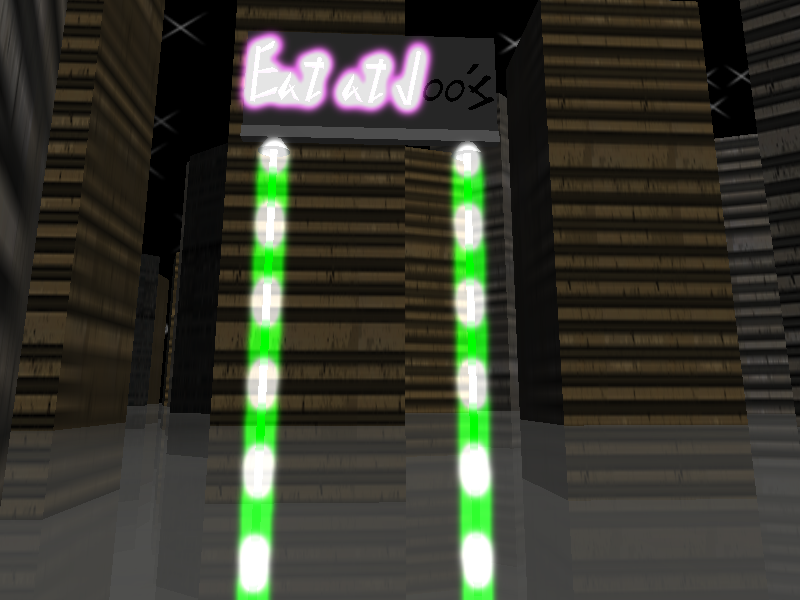Assets

You can make the futuristic scene as complicated as you want, but I'm going to keep it sorta simple.  What we'll have is a bunch of .obj models for the following things
• Skybox (day and night)
• Buildings
• Base of the billboard
• Supports for the billboard (eventually our anti-gravity beams)
Of course we'll have various textures being used.  Use whatever pleases you for textures, but the one's for specific shaders that we'll make will be discussed when we will make use of them.

Non-GLSL

In case you need to get an idea of how the scene looks like before we start applying any shader work, here are a couple screen shots of day and night.This is the first run that I came up with so far.  Now I have something to work with.  Here we have kinda funny textured buildings.  At the bottom we have a fog.  What we'll do for now is just use multi-texturing and animate it by modifying the texture coordinates over time.  The letters on the sign are an actual .obj model and not a texture.

So the first thing we'll do is having something that is kinda cool and useable during the day time.

Daytime GLSL Standard Stuff

How about some basic stuff?  Directonal lighting with texture mapping?  This is a basic ability and is covered all over the web.  We'll do per-fragment lighting, and in this case we will just do simple diffuse lighting.

For lighting we need to send from the vertex shader to the fragment shader:
• Transformed vertex
• Light Direction with respect to the eye
• Diffuse and ambient colors after combining the diffuse and ambient contributions of the materials and light
For texture mapping we need:
• The application to send which texture unit the color map is bound to
• The vertex shader to pass the texture coordinates to the fragment shader
Below is what our vertex shader looks like for the basic set of things.  As you can see, there are a lot of things that come in for free (i.e. don't have to be setup as special uniforms), such as the light source position, diffuse and ambient colors.  This is assuming you've setup the proper attributes in the application as you would do with a standard OpenGL application without using shaders.

varying vec3 tnorm;    // our transformed normal
varying vec3 lightdir; // the light direction vector
varying vec4 diffuse;
varying vec4 ambient;

void main(void)
{

// get the vertex in modelview space
vec4 tvec = gl_ModelViewMatrix * gl_Vertex;
// light direction in eye space
lightdir = normalize( vec3( gl_LightSource.position - tvec ) );
// our fully transformed vertex
tvec = gl_ProjectionMatrix * tvec;

// our normalized, transformed normal
tnorm = normalize( gl_NormalMatrix * gl_Normal );

// calculate what our diffuse and ambient colors would be
// if the light where dead on
// Kd = Ld * Md
// Ka = (La * Ma) + (Ga * Ma)
diffuse = gl_FrontMaterial.diffuse * gl_LightSource.diffuse;
ambient = gl_FrontMaterial.ambient * gl_LightSource.ambient;
ambient += gl_LightModel.ambient * gl_FrontMaterial.ambient;

// pass our texture coordinates gained from glTexCoord2f calls
gl_TexCoord = gl_MultiTexCoord0;

// finish vertex shader
gl_Position = tvec;
}

Once we get that necessary data, and we have setup a uniform for our sampler2D that we will use to get the color map, we can finally fill in the color of our object.

Now in my scene I have some things that don't use textures, such as the billboard itself.  I also have parts where I don't want lighting applied and just want the texture applied as normal, such as the skybox and night skybox.  I'll need to add integer flags to indicate if some calculations should occur.  Here is the fragment shader to do so, with diffuse lighting calculated and textures used, if the flags are turned on.   Note: You can use glUniform1i to assign a value of 0 or 1 to a uniform boolean.

uniform bool dolighting; // do we do lighting calculations?
uniform bool usetexture; // do we use the texture color?
uniform sampler2D colormap; // our color map texture

varying vec3 tnorm
varying vec3 lightdir;
varying vec4 diffuse;
varying vec4 ambient;

void main(void)
{
// make sure we have a normalized normal
vec3 n = normalize(t norm);

// assign a color of white in case we don't do lighting
vec4 lightColor = vec4( 1.0, 1.0, 1.0, 1.0 );
if( dolighting )
{
lightColor = ambient;
// perform standard diffuse lighting calculations
//using dot product
float ndotl = max( dot( n, lightdir), 0.0 );
if( ndotl > 0.0 )
{
lightColor += diffuse * ndotl;
}
}

// assign a color of white in case we don't use color map
vec4 texColor = vec4( 1.0, 1.0, 1.0, 1.0 );
if( usetexture )
texColor = texture2D( colormap, gl_TexCoord.st );

// combine the colors to produce our final color
gl_FragColor = texColor * lightColor;
}

So how are we using it in the daytime scene?  Here is psuedocode to describe how we're using it for drawing the billboard, buildings and sky/night box.

1. Bind the shader
2. Set "usetexture" to 0 and "dolighting" to 1
3. Draw our billboard related objects (text, base, poles)
4. Set "usetexture" and "dolighting" to 1
5. Draw our buildings
6. Set "usetexture" to 1 and "dolighting" to 0
7. Draw our sky/night box and draw our semi-transparent cloud plane
What a useful shader!!!  Here's a screen shot where you can see the psuedocode in action.  Looks about the same as fix functionality, minus specular highlights since we aren't calculating it.... Yet.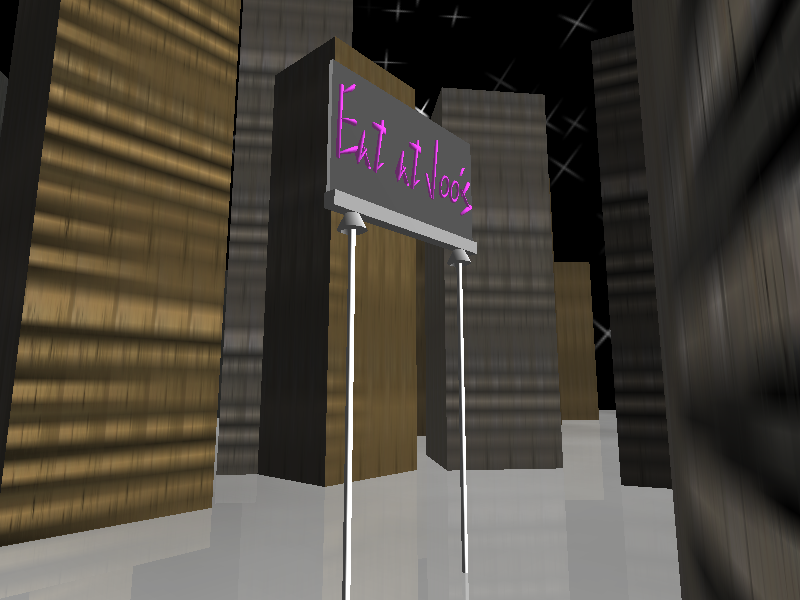Daytime GLSL Pulsating Beam

So let's add some unusual spice to our scene for the day time.  How about making the poles that are holding up the billboard look sci-fi like.  What we'll do is have pulsating colors shooting out from the thruster looking things at the base of the billboard.  Let's pick the color green.  Why?  Because nothing else in the scene is green, and that's the color of Luc's lightsaber in Return of the Jedi.  So our goal is to have spurts of light or color shooting down, and have the pole be transparent in between the bursts.  Cool!

How are we going about doing this?  Well we can use a sine function to be a periodic function that would describe how much light we would have at a spot.  The nice thing is that we'll have some smoothness to this compared to using a step function that is either on or off.

So where are we going to do most of our work?  Since we're modifying colors and discarding fragments, we should be doing most of our work in the fragment shader.  What we'll do first is figure out how our fragment shader should look like.  This will then tell us what our vertex shader needs to send in as a "varying" data and what our application code should send in as "uniforms" or "attributes."

First, we need to be able to know where we are vertically, so we'll send in a y-position from the vertex shader.  Also, we'll need something to let us know what time it is, and that will have to be a uniform coming in.
So far this is what our fragment shader looks like.

varying float y;
uniform float time;

void main(void)
{
// store the result of our moving sine function,
// and remove negative values from the picture
float intensity;
intensity = sin( y + time );

// draw our color as a non transparent shade of green
gl_FragColor = vec4( 0.0, intensity, 0.0, 1.0);
}
So what does our vertex shader look like right now?  Simple enough, just get the y-position from the incoming gl_Vertex and pass it on.  The value will get interpolated later on.

varying float y;

void main(void)
{
// get our y position
y = gl_Vertex.y;

// transform our vertex
gl_Position = gl_ModelViewProjectionMatrix * gl_Vertex;
}

Here's the results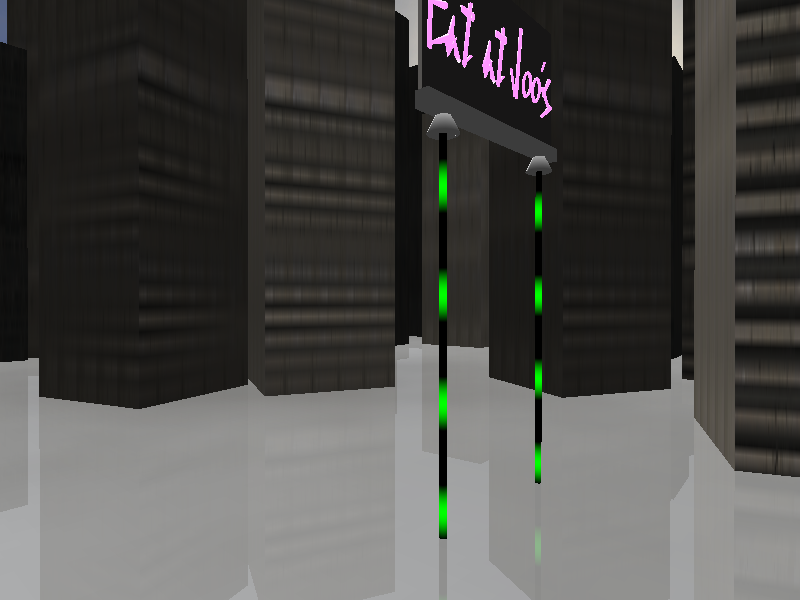Notice the black parts.  That's something we should get rid of.  Here's where we add the discard call.  All we have to do is check if our intensity value is less than or equal to some value.  What shall our value be?  If we use zero then we will still have the somewhat dark parts of green.  Light can't be dark so let's use the value of 0.5.  Our new fragment shader looks like this.

varying float y;
uniform float time;

void main(void)
{
// store the result of our moving sine function,
// and remove negative values from the picture
float intensity;
intensity = sin( y + time );

// draw our color as a non transparent shade of green
// and get rid of black
if( intensity <= 0.5 )
else
gl_FragColor = vec4( 0.0, intensity, 0.0, 1.0);
}

Below is what we get.  It's an improvement, but notice something funny?  You can see a curving on the bottom green bursts.  That's because I've enabled back face culling.  If I get disable that it will help, but here's a fundamental problem with this effect.  Can you guess?  If we look straight down the y-axis we won't see anything because there is no face being filled in that is above the fog!!!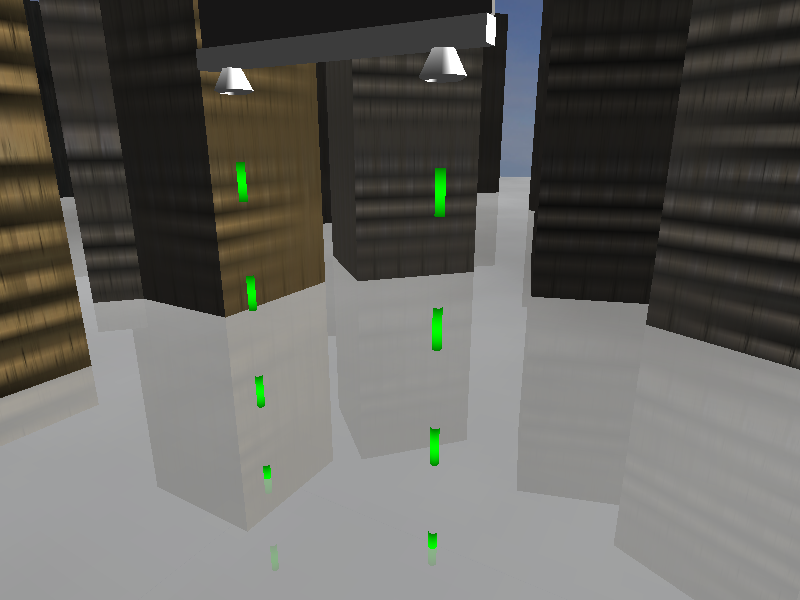Let's re-think about how to do this pulse affect without having to do weird stuff in the application end.  How about we have the color vary between green and white!  Now we don't have to do a discard and it will probably really tell some flying car not to come near!  In order to do that we'll use the intensity value to modify our red and blue contributions and keep our green channel at 1.0 all the time.

Let's also add a scale factor to increase the number of bursts we have per second.  So here is our third version of our first day time fragment shader.  Feel free to play with the RATE_SCALE macro.  In fact, it would be a good exercise to make it an incoming uniform that you can change with some key bindgs!

varying float y;
uniform float time;

#define RATE_SCALE 5.0

void main(void)
{
float intensity;
intensity = sin( y + (time * RATE_SCALE));
intensity = clamp( intensity, 0.0, 1.0);

// vary color from white to green by varying red & blue
gl_FragColor = vec4( intensity, 1.0, intensity, 1.0);
}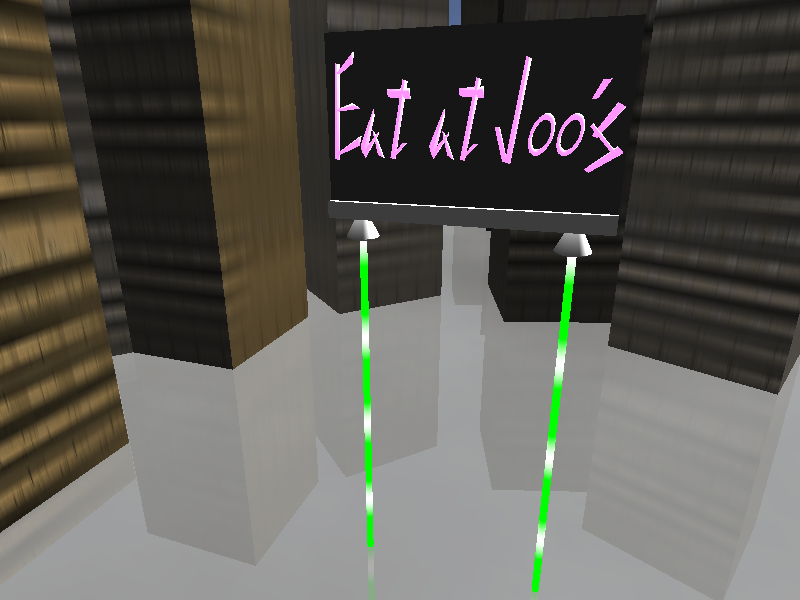Now let's move onto doing something at night.

Night-time GLSL Glowy Effect

Now we're going to kick up the difficulty a bit here.  What we're going to do is make the "Eat at Joo's" sign glow, as if it were emmitting light.  We'll also apply the affect to the energy poles.  In order to do this we're going to make several off-screen framebuffer objects, and perform render to texture operations with different shaders in affect.  Here's our attack pattern:
1. Render the scene to a texture in the normal way we were before.  This includes our pulsating energy pole.
2. We render our scene again, but this time to a texture with that is 1/4 the size (i.e. both dimensions are half).  Also we only render the things that will glow, which are our energy poles and billboard text
3. We bind a blur shader
4. We render our results of step 2 to another FBO using the blur shader
5. We render our results of step 4 to the same FBO in step 2 and use the blur shader
6. We repeat step 4 and 5 until we have blurred the image enough
7. We use a shader to combine the results of step 1 and step 6 to the default frame buffer.
At this point I will assume you know how to setup framebuffer objects in OpenGL.  It is recommended that you use textures for both color and depth attachments.  You will see why after we've gone through making the glow effect the first time.

For step 1 and 2 we use the same shaders as we have before.  Again, for step two we only apply the things that we will want to blur.  In this case we will blur the energy pole and the billboard text.

For step each of the blurring steps we render a screen sized quad with our 1/4 size texture applied.  The first blur pass we use have an un-blurred image.  Below is the original image and then the image we would expect to see after doing nine passes with our blur filter.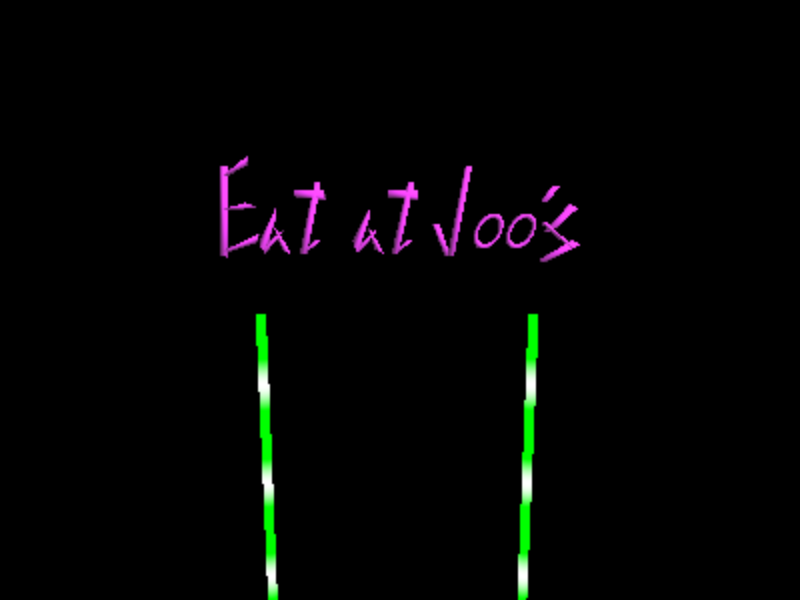No Blur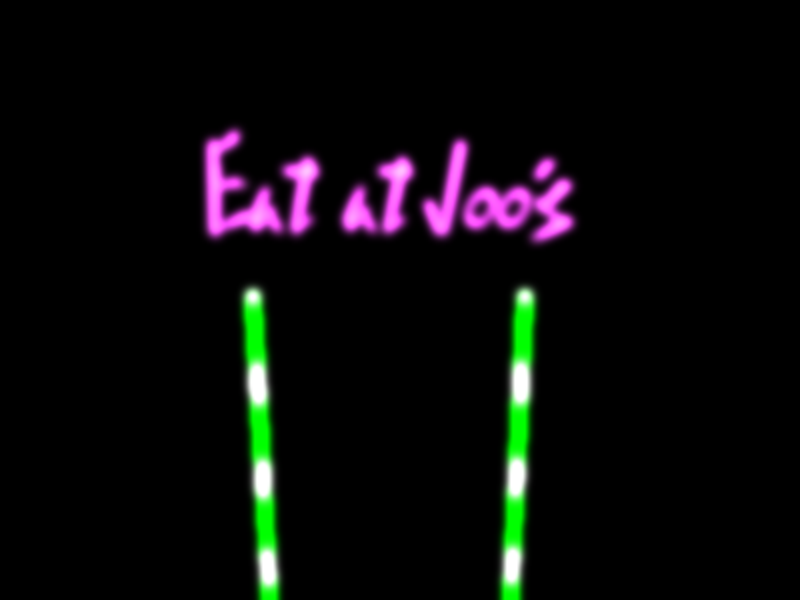After nine passes

Our vertex shader is very simple as it will just transform our vertex and pass our texture coordinates to our fragment shader.

void main(void)
{
gl_TexCoord = gl_MultiTexCoord0;
gl_Position = ftransform();
}

Our fragment shader is a bit more complicated.  Because we are working with an image, and applying a blur affect, we are going to need a kernel.  This is going to be basic convolution.  We are going to sum colors of neighboring pixels in order to blur.  Here we will use a 3x3 box filter, which is basically a matrix full of 1's.

1.0   1.0   1.0
1.0   1.0   1.0
1.0   1.0   .10

Our Box Filter Kernel

Remember that the sum of all elements in a kernel should equal 1.0.  This means that if we sum our elements then our normalization factor would be the inverse of that sum.  In this case, it would be 1.0 / 9.0.

Of course, we can't apply convolution on an image if we don't have an image source.  This means our fragment shader needs a uniform texture sampler.  Because I am using a rectangular texture, I will use samplerRect.  NOTE: If you have a Mac, up to OS X 10.4.6, samplerRect is not supported.  Hopefully it will be supported in the future.

// our source image
uniform samplerRect colormap;
Our fragment shader will, for every pixel, apply the filter by sampling adjacent pixels.   So here we're going to try something new.  We're going to use a for-loop, and go through an array of kernel elements and array of texture coordinate offsets, which will let us access adjacent pixels.  We also want to make sure that the brighter parts of the image get brighter, giving us "hot spots."  Take a closer look at the letter "t" in our expected results after nine passes.  There's a slight hot spot on the upper part of the letter.  In order to do this we will scale the sampled color by a factor greater than one.  The more passes we perform, the brighter the image will be.

// our 3 x 3 kernel
float kernel;
// our normalization factor for our kernel
float normfac = 1.0 / 9.0;
// our texture access offset vectors
vec2 offset;

// our source image
uniform samplerRect colormap;

void main(void)
{
int i;
vec4 colorsum = vec4(0.0, 0.0, 0.0, 0.0);

// setup our box filter kernel
kernel = 1.0; kernel = 1.0; kernel = 1.0;
kernel = 1.0; kernel = 1.0; kernel = 1.0;
kernel = 1.0; kernel = 1.0; kernel = 1.0;

// setup our offsets, go per row
// row 0
offset = vec2( -1.0, -1.0 );
offset = vec2( 0.0, -1.0 );
offset = vec2( 1.0, -1.0 );
// row 1
offset = vec2( -1.0, 0.0 );
offset = vec2( 0.0, 0.0 );
offset = vec2( 1.0, 0.0 );
// row 2
offset = vec2( -1.0, 1.0 );
offset = vec2( 0.0, 1.0 );
offset = vec2( 1.0, 1.0 );

// go through sample
for( i = 0; i < 9; i++)
{
// sample with offset coordinate
vec4 sample;
sample = textureRect( colormap, gl_TexCoord.st + offset[i]);
// scale the sample
sample = sample * 1.3;
// aggregate color, with weight from kernel
sum += sample * kernel[i] * normfac;
}

gl_FragColor = sum;
}

Combining Base and Blur

Now that we have our blurred image, we need to combine it with the base image that we made in step 1.  This will complete the glow effect, for the most part.

Here we will put the base image in texture unit 0 and the final blur image in texture unit 1.  Our vertex shader for the combiner will be the same as our blur vertex shader, with one modification.  We will assign a value to gl_TexCoord to take into account that our blur texture is 1/4 the size of our base texture.

void main(void)
{
gl_TexCoord = gl_MultiTexCoord0;
gl_TexCoord = gl_MultiTexCoord0 * 0.5;
gl_Position = ftransform();
}

Our combining fragment shader is also pretty simple.  Here we just sample our color maps and sum the colors.  Before summing the colors, we apply a blending factor to the sampled blur color.  This will give the illusion of some transparency.  Here I decide to incorporate with a blend factor of 0.5.

uniform samplerRect basemap;
uniform samplerRect blurmap;

float blend_fac = 0.5;

void main(void)
{
// get our base and blur colors
vec4 baseclr = textureRect( basemap, gl_TexCoord.st );
vec4 blurclr = textureRect( blurmap, gl_TexCoord.st );

// apply blend amount
blurclr = blurclr * blend_fac;

// sum up
gl_FragColor = baseclr + blurclr;
}
How come we can just add the colors up?  Simple, we're trying to make a glow.  This means our fragments should be brighter.  On top of that, most our blurmap is black, thus most of the time we sum with 0.0.  Here's what we get when we run this shader on the last pass, to the default frame buffer.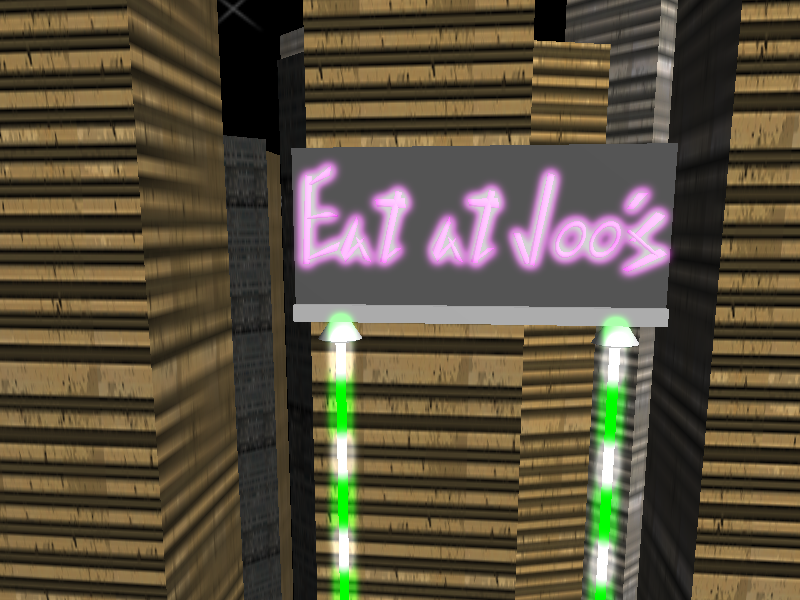Do you notice something peculiar about the image.  Look at the top of the energy pole.  There's light outside the little "thruster-like" things at the base of the billboard.  Here's another view to give you a better idea of the problem.It should be obvious.  We aren't checking the difference in depth.  We're just blending colors together.  How are we gonna slove this problem???!!!

Minimizing Depth Error with Glow Effect

Here's where we solve the problem with the blurred image completely ignoring the depth of the scene when combining the blurred and base textures.

We have to draw everything.  But here's the trick.  We don't do lighting on the things that aren't supposed to be glowing.  We also don't apply textures.  What does that give us?  Black.  On the worst case we'll have some color leakage.  Especially if we add more blur passes.  Here are three images that describe what the difference will be when we're done.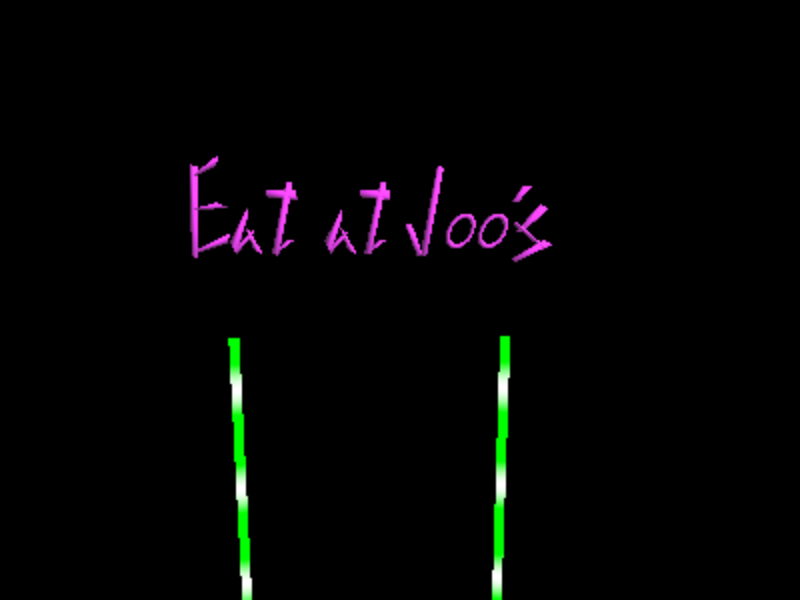Looks about the same as before.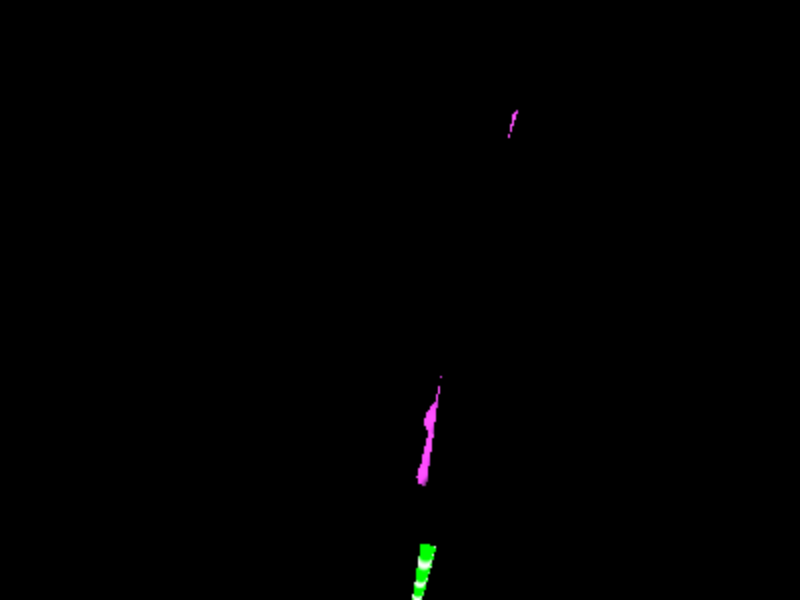Here some of the text has been obscured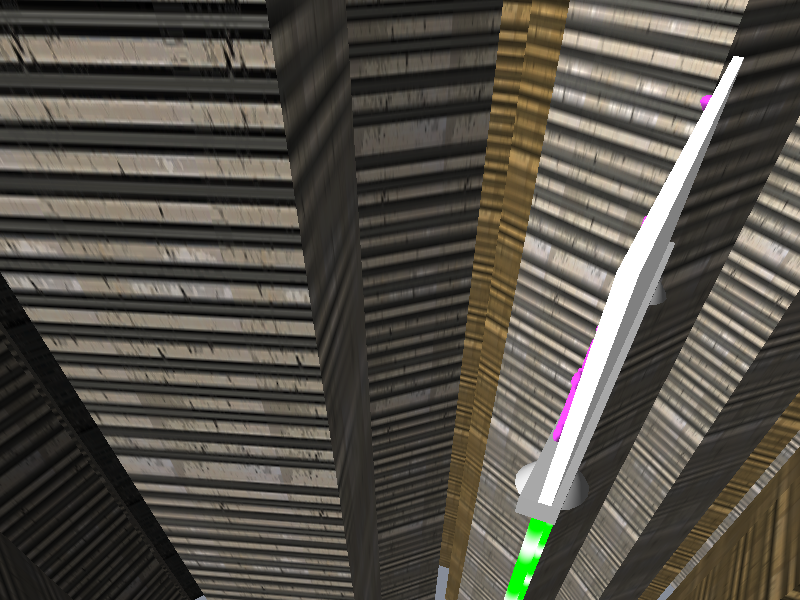A similar view to show how the text is obscured.

We will make our changes to our standard fragment shader, by adding another uniform bool called "makeblack" that, when enabled, will ignore lighting and texture calculations and force the fragment color to be black.

uniform bool dolighting;
uniform bool usetexture;
uniform bool makeblack; // overrides all other color calculations

...

void main(void)
{
// do lighting
...
// do texture mapping
...

// determine final color
if( makeblack )
gl_FragColor = vec4( 0.0, 0.0, 0.0, 1.0);
else
gl_FragColor = texColor * lightColor;
}While we are here, we can make some modifications to our application code to have a more distinct night & day.  For the day time, we can still apply our glow affect at a reduced amount by having a lower number of blur passes.  For the night we can lower our light sources diffuse and ambient term to produce a generally darker scene.  Coupling that with more blur passes will produce a relatively brighter neon glow affect.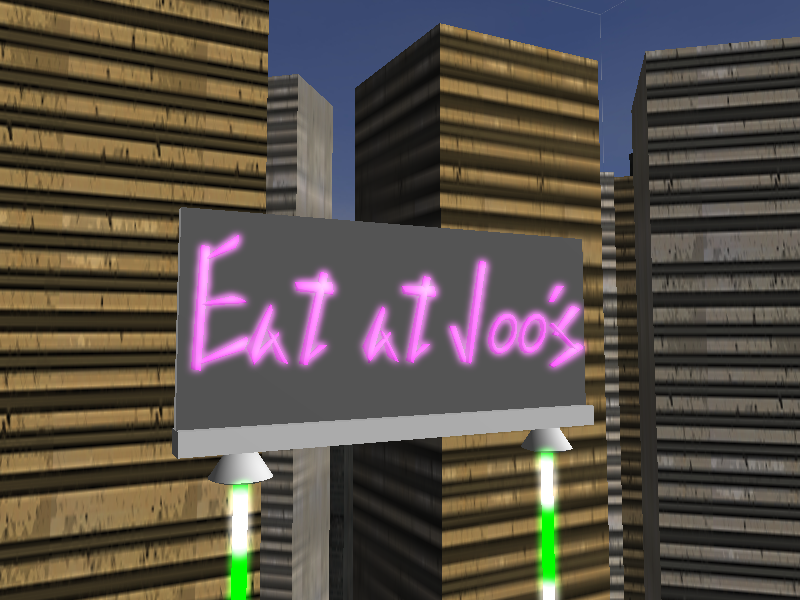Day time, with glow affect.  5 blur passes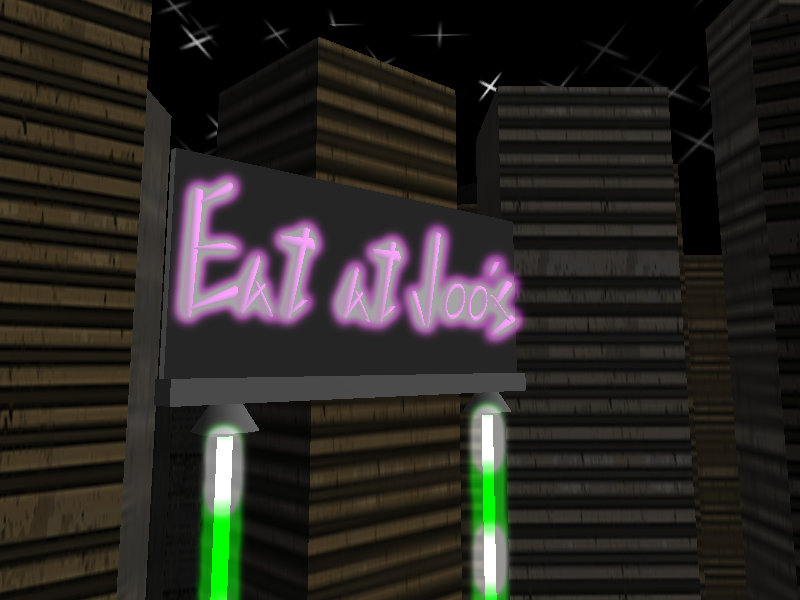Night time with glow affect.  13 passes.

Extras

Letter Animation

Let's add some little extras to our billboard.  If the letters of the sign were made into seperate models, we can easily animate the sign in our application code.  We would do this by adding a delay of 1 second to counting a number cycling through 0 to (N + 1), where N is the number of characters.  If our letter in our array is less than the number we light it up.  Lighting it up is basically making use of the "makeblack" uniform that we can turn on or off.   In my case, I have broken down my sign into nine letters, so N = 9.  Here is the code for making this happen

// Have a Global LetterFrame and LetterDelay variables
int LetterFrame;
float LetterDelay;

...

// In a function that updates the scene given some change in time (deltaTime)
LetterDelay -= deltaTime;
if( LetterDelay < 0 )
{
// update our animation frame
LetterFrame = (LetterFrame + 1) % 10;
// setup our delay
LetterDelay = .5f; // half a second
}

...

// In our draw function ...
for( int i = 0; i < 9; i++)
{
// if our index is less than the frame then don't make it black
if( i < LetterFrame )
{
set_uniform1i( StandardShaderEffect, "makeblack", 0);
}
else
{
set_uniform1i( StandardShaderEffect, "makeblack", 1);
}

// draw out the letter at our current index
draw_letter(i);
}

...
This will effectively animate the letters so that our letters will light up from left to right.  Here is a screenshot with glow affect being applied to only some of the letters at night.Fog with more Umph!

We'll ditch the fake fog we have and do some fake fog affects in our shaders. What we'll do is have a whole bunch of horizontal slices, and use three low resolution fog textures (see below).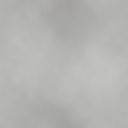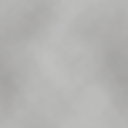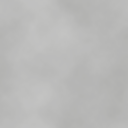The three textures are applied to each slice using OpenGL's multitexturing.  We modify the texture coordinates that we will use in the application side the normal way you would animate a texture on a surface.

S_coord += deltaTime * deltaS;
T_coord += deltaTime * deltaT;

At each slice we apply our shader that will modify how the animation will occur.  The vertex shader will just pass the texture coordinates we need through, and perform a fixed function transformation call.  It will also send the incoming y-position of the vertex, which will let us know what height we are in the scene.

varying float height;

void main(void)
{
height = gl_Vertex.y;

gl_TexCoord = gl_MultiTexCoord0;
gl_TexCoord = gl_MultiTexCoord1;
gl_TexCoord = gl_MultiTexCoord2;

gl_Position = ftransform();
}
Our fragment shader will use the incoming varying with three texture samplers and an incoming uniform time variable.  Then we will use a sine function to modify how we will scale the incoming texture coordinates.  In the sine function we will use the incoming time and height so that we will have different shifts per height.  This is so our fog will have a little bit of body to it.  Here we will take the absolute value of the sine curve because I wanted the fog to be moving in a general direction, instead of moving back and forth.  In essence, it's like a rolling mass of fog.  I also scaled my time factor by 1/30 to slow down the fog's movement.

uniform sampler2D tex0;
uniform sampler2D tex1;
uniform sampler2D tex2;

uniform float time;

varying float height;

void main(void)
{
// determine how much we will scale our tex coords
float s0, s1, s2;
s0 = abs(sin(time / 30.0 + height));
s1 = abs(sin(time / 30.0 + 1.0 + height));
s2 = abs(sin(time / 30.0 + 2.0 + height));

// get the colors
vec4 c0, c1, c2;
c0 = texture2D( tex0, gl_TexCoord.st * s0);
c1 = texture2D( tex1, gl_TexCoord.st * s1);
c2 = texture2D( tex1, gl_TexCoord.st * s2);

// modify the color by the height, up to a certain point
float cscale = max( abs(height) / 5.0, 1.0);
c0 *= cscale;
c1 *= cscale;
c2 *= cscale;

// blend
gl_FragColor = c0 * c1 * c2;
}

In the end we get a sorta thick looking animated fog.  I'ts a subtle effect, and really cheap.  One of the weaknesses is that if you have the horizontal slice cut into something that is of a different color than the textures, it becomes very appearent that it's just a 2D slice.  Not something volumetric.  I should note that this object is not rendered when setting up the depth corrected glow filter.  This is because the light from the energy poles should go through the fog.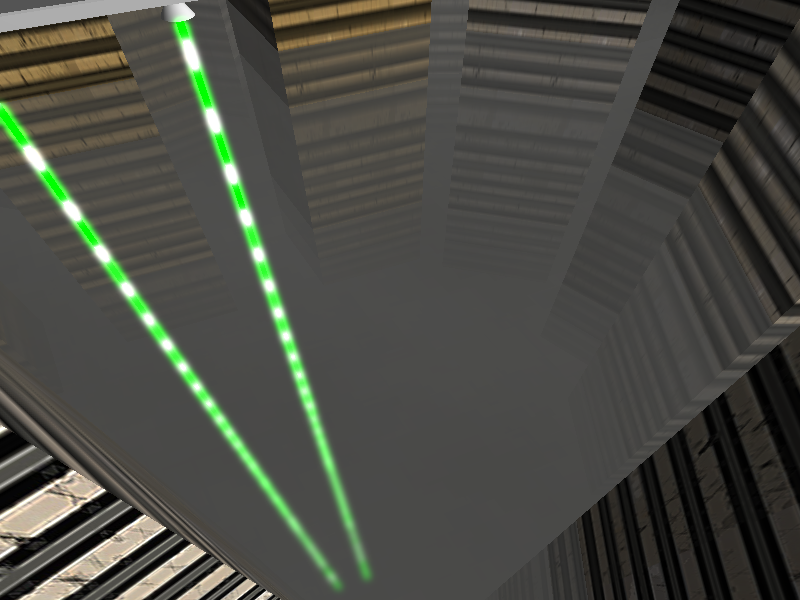So here we are.  Done with our Billboard of the Future.  Hope you enjoyed the show!! And now for some parting messages.

Here you can download the source code to this project.  arun_final.tar.gz

It has been tested on SuSe Linux 10.0 with a GeForce 7800 GT, and on Redhat 7.3 with a GeForce 5000 series card.   You will need to modify the makefile in the ./src directory.  There are two main sections to that makefile, one for the Mac and one for Linux (both x86_64 and 32-bit).  The Mac part of the makefile is there so I can test updates to Mac OS X, to quickly see if certain features have been implemented.

Running the program.  The program is called "final".  Go to the root subdirectory of the project and just type ./final to run.  Controls are simple.
W - move forward
S - move backward
A - strafe left
D - strafe right

Mouse - look around

I - Toggle Day/Night mode
C - Take a screen shot (automatically saved to default_fb.png)
If you have any questions please feel free to contact me at  " arun dot g dot rao at gmail dot com." And for those of you who don't like to read that kind of thing you can mail me here: arun.g.rao@gmail.com